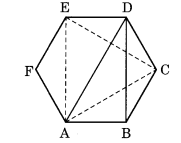NCERT Solutions for Class 6 Maths Chapter 5 Understanding Elementary Shapes Ex 5.8

# NCERT Solutions for Class 6 Maths Chapter 5 Understanding Elementary Shapes Ex 5.8

## NCERT Solutions for Class 6 Maths Chapter 5 Understanding Elementary Shapes Ex 5.8

NCERT Solutions for Class 6 Maths Chapter 5 Understanding Elementary Shapes Ex 5.8 are the part of NCERT Solutions for Class 6 Maths. Here you can find the NCERT Solutions for Class 6 Maths Chapter 5 Understanding Elementary Shapes Ex 5.8.

### Ex 5.8 Class 6 Maths Question 1.

Examine whether the following are polygons. If any one among them is not, say why?

Solution:

We know that a polygon is a closed figure bounded by line segments.
(a) The given figure is not closed. Therefore, it is not a polygon.
(b) The given figure is a polygon because it closed and bounded by 6 line segments.
(c) The given figure is not a polygon because it is not enclosed by line segments.
(d) The given figure is not a polygon because it is enclosed by an arc and two line segments.

### Ex 5.8 Class 6 Maths Question 2.

Make two more examples of each of these.

Solution:
(a) This is a quadrilateral. Examples:

(b) This is a triangle. Examples:

### Ex 5.8 Class 6 Maths Question 3.

Draw a rough sketch of a regular hexagon. Connecting any three of its vertices, draw a triangle. Identify the type of the triangle you have drawn.

Solution:
ABCDEF is a rough sketch of a regular hexagon. If we join any three vertices like D, A and B, we get a scalene triangle ABD.But if we join the alternate vertices, we get an equilateral triangle ACE.

### Ex 5.8 Class 6 Maths Question 4.

Draw a rough sketch of a regular octagon. (Using squared paper if you wish). Draw a rectangle by joining exactly four of the vertices of the octagon.

Solution:
ABCDEFGH is a rough sketch of a regular octagon. CDGH is a rectangle formed by joining the four vertices of the given octagon.

### Ex 5.8 Class 6 Maths Question 5.

A diagonal is a line segment that joins any two vertices of the polygon and is not a side of the polygon. Draw a rough sketch of a pentagon and draw its diagonals.

Solution:
ABCDE is the rough sketch of a pentagon. By joining any two vertices, we get the following diagonals.

You can also like these:

NCERT Solutions for Maths Class 7

NCERT Solutions for Maths Class 8

NCERT Solutions for Maths Class 9

NCERT Solutions for Maths Class 10

NCERT Solutions for Maths Class 11

NCERT Solutions for Maths Class 12